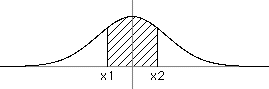### Problem Statement

This problem contains HTML superscripts and images which will not display correctly for plugin users

Given two real numbers x1 and x2, calculate an approximation to the integral of e-x^2 evaluated between the limits from x1 to x2, which is accurate to the nearest 0.00001. Return the answer in a String, as a fixed point number with exactly five digits to the right of the decimal point and exactly one digit to the left of the decimal point.

For example: x1 = -0.5 and x2 = 0.5 returns "0.92256"

### Definition

 Class: NumericalIntegral Method: integrate Parameters: double, double Returns: String Method signature: String integrate(double x1, double x2) (be sure your method is public)

### Notes

-
• e-x^2 can be calculated in C++ with exp(-x*x) in math.h.
• e-x^2 can be calculated in C# with Math.Exp(-x*x). The Math class is in the System namespace.
• e-x^2 can be calculated in Java with Math.exp(-x*x).
• e-x^2 can be calculated in Visual Basic with Exp(-x*x) in the System.Math namespace.
--The integral of a function is the area inside the closed figure formed by (on the top) the function between the limits of x=x1 and x=x2, (on the sides) vertical line segments at x=x1 and x=x2, and (on the bottom) the portion of the x axis between x=x1 and x=x2. This is shown by the shaded area above (the graph shows the function we are integrating, e-x^2).
-The integral of e-x^2 is known to have no closed form, so don't waste time looking in a table of integrals for an exact formula.
-Because of the 2e-6 constraint, about 40% of randomly chosen x1 and x2 values will be too close to a possible rounding error and will be rejected. This is not an error. It gives you more room for numerical errors.

### Constraints

-x1 will be less than x2.
-x2-x1 will be between 0.00001 and 1.00000 inclusive.
-x1 will be between -10.0 and 10.0 inclusive.
-x2 will be between -10.0 and 10.0 inclusive.
-To avoid rounding errors the inputs x1 and x2 must be chosen so that the answer is not within 2e-6 of 0.000005 + a multiple of 0.00001

### Examples

0)

 `-0.5` `0.5`
`Returns: "0.92256"`
 The example from above. This is the largest possible answer given the constraints of this problem.
1)

 `0.0` `0.1`
`Returns: "0.09967"`
2)

 `-9.0001` `-9.0`
`Returns: "0.00000"`
 Values are very small out here.
3)

 `2.71828183` `3.14159265`
`Returns: "0.00010"`

#### Problem url:

http://www.topcoder.com/stat?c=problem_statement&pm=2375

#### Problem stats url:

http://www.topcoder.com/tc?module=ProblemDetail&rd=4755&pm=2375

Rustyoldman

#### Testers:

lbackstrom , brett1479

#### Problem categories:

Advanced Math, String Manipulation